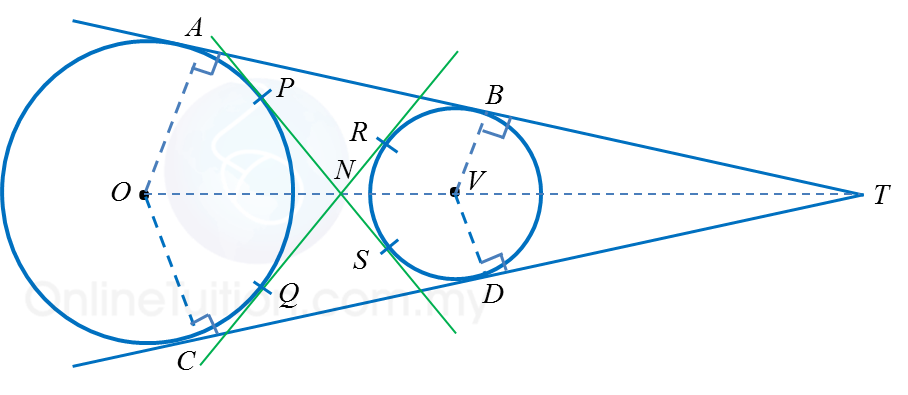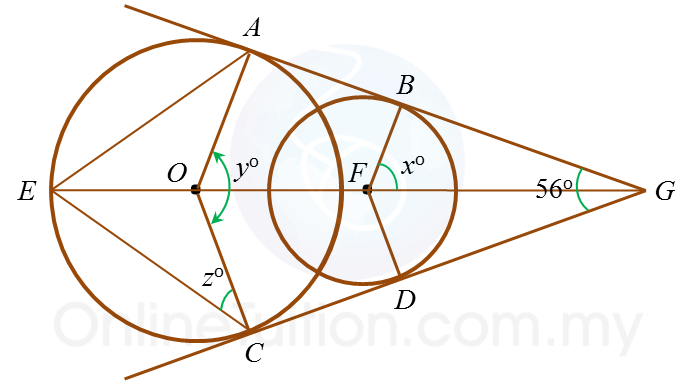# 6.5.2 Common Tangents (II)

6.5.1 Common Tangents (II)

3. Do not intersect
(a) Circles of the same sizeNumber of common tangents Properties of common tangents Four common tangents: AB, CD, PS and RQ AB = CD = OV PS = RQ AB // OV // CD

(b) Circles of different sizesNumber of common tangents Properties of common tangents Four common tangents: AB, CD, PS and RQ AB = CD BT = DT PS = RQ OA // VB OC // VD

Example 1:In the diagram, ABG and CDG are two common tangents to the circle with centres O and F respectively. Find the values of
(a) x,  (b) y,  (c) z.

Solution:
(a)
In ∆ BFG,
angle BFG = ½ × 56o = 28o
angle FBG = 90o ← (tangent is 90o to radius)
x+ 28o + 90o = 180o
x= 180o – 118o
x= 62o
x = 62

(b)
angle AOF = xo = 62← (AO// BF)
y= 2 × 62o
y= 124o
y = 124

(c)
Exterior angle of AOC = 360o – 124o = 236o
Angle EOC = ½ × 236o = 118o
z= (180o – 118o) × ½  ← (∆ EOC is isosceles triangle, angle OEC = angle OCE)
z= 31o
z = 31